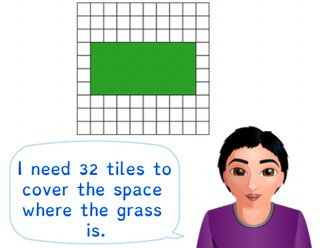Determining simple area using squares

# Determining simple area using squares

Students learn to determine simple area using squares.

No account needed.8,000 schools use Gynzy92,000 teachers use Gynzy1,600,000 students use Gynzy

## General

The students learn how they can determine the area of a shape using squares.

## Standards

CCSS.Math.Content.3.MD.C.5/6

## Learning objective

Students will be able to determine area using squares.

## Introduction

There is a number on the interactive whiteboard. Explain that the correct number of cookies must be dragged to the number. For this you can use the package of 10 cookies and the single cookie. Repeat this exercise with the sunglasses.

## Instruction

Ask if the students can explain what area is. Say that area is the surface of a shape. There are different ways in which you can determine how large an area is. First discuss this with a rectangular shape. In this case, you can count the colored squares inside the shape. You can also use the multiplication tables. For this you do the length × the width. Explain that when a shape isn't a square or rectangle, you can still use the multiplication tables. For these shapes, however, you need to pay attention to the squares that aren't colored, and subtract these from the total. Show two different shapes and ask what the area is. Erase the covers to show what the answer is and ask what the students notice. Explain that shapes the look different can still have the same area. After this, show a number of shapes for which the students must determine the area. Ask the students how they determined the area of the different shapes. You can also determine the area of a shape with diagonal lines. If the line goes exactly through the middle of a square then that makes halves of a square. You add the half squares together to make whole squares. Also have the students practice determining the area of the following shapes with diagonal lines.

To check whether the students understanding how to determine area, you can ask them the following question:
- How do you determine the area of a shape?

## Quiz

The students test their understanding of determining area using squares through ten exercises. In each of these exercises, they must determine the area of a square or rectangle. They can do this by counting the squares in the shape, or by multiplying the length by the width.

## Closing

You discuss once again with the students how it is useful to be able to determine area using squares, because then you can quickly determine what the area of the surface is. Have the students work in pairs to practice determining area using squares. Hand out graphing paper and have each student draw a shape. The pairs trade drawings and must determine the area of their partner's shape.

## Teaching tips

When students have difficulty with determining area using squares, have them draw a line through the shape, using it to count each of the squares that are in the shape. After this they can practice multiplying the length and the width. Emphasize that for shapes that aren't a square or rectangle, they must subtract the missing squares that aren't colored in from the total.

## Instruction materials

Graphing paper

### The online teaching platform for interactive whiteboards and displays in schools

• Save time building lessons

• Manage the classroom more efficiently

• Increase student engagement